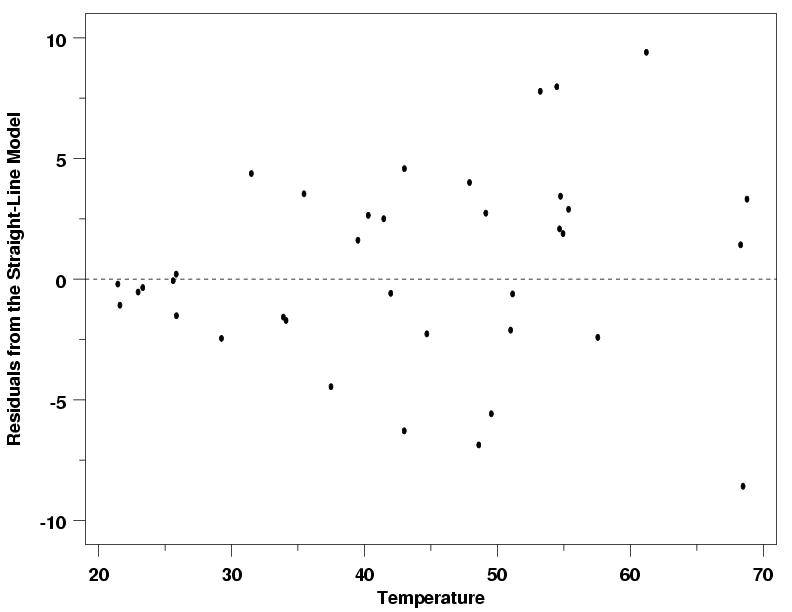# Residual Plot: Definition and Examples

Regression Analysis > Residual Plot

## What is a Residual Plot?

Watch the video for an overview and several residual plot examples:

A residual value is a measure of how much a regression line vertically misses a data point. Regression lines are the best fit of a set of data. You can think of the lines as averages; a few data points will fit the line and others will miss. A residual plot has the Residual Values on the vertical axis; the horizontal axis displays the independent variable.A residual plot is typically used to find problems with regression. Some data sets are not good candidates for regression, including:

• Heteroscedastic data (points at widely varying distances from the line).
• Data that is non-linearly associated.
• Data sets with outliers.

These problems are more easily seen with a residual plot than by looking at a plot of the original data set. Ideally, residual values should be equally and randomly spaced around the horizontal axis.

## Examples

If your plot looks like any of the following images, then your data set is probably not a good fit for regression.The outlier is clearly apparent in this residual plot. Image: PSU.edu

The residual plot itself doesn’t have a predictive value (it isn’t a regression line), so if you look at your plot of residuals and you can predict residual values that aren’t showing, that’s a sign you need to rethink your model. For example, in the image above, the quadratic function enables you to predict where other data points might fall. For a residual plot, that’s not a good thing. If your plot indicates a problem, there can be several reasons why regression isn’t suitable. It doesn’t always mean throwing out your model completely, it could be something simple, like:

• Missing higher-order variable terms that explain a non-linear pattern.
• Missing interaction between terms in your existing model.
• Missing variables.

## Residual Plot: References

Beyer, W. H. CRC Standard Mathematical Tables, 31st ed. Boca Raton, FL: CRC Press, pp. 536 and 571, 2002.
Agresti A. (1990) Categorical Data Analysis. John Wiley and Sons, New York.
Klein, G. (2013). The Cartoon Introduction to Statistics. Hill & Wamg.
Vogt, W.P. (2005). Dictionary of Statistics & Methodology: A Nontechnical Guide for the Social Sciences. SAGE.

CITE THIS AS:
Stephanie Glen. "Residual Plot: Definition and Examples" From StatisticsHowTo.com: Elementary Statistics for the rest of us! https://www.statisticshowto.com/residual-plot/